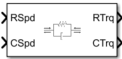# Torsional Compliance

Parallel spring-damper

• Library:
• Powertrain Blockset / Drivetrain / Couplings

Vehicle Dynamics Blockset / Powertrain / Drivetrain / Couplings

•## Description

The Torsional Compliance block implements a parallel spring-damper to couple two rotating driveshafts. The block uses the driveshaft angular velocities, torsional stiffness, and torsional damping to determine the torques.

$\begin{array}{l}{T}_{R}=-\left({\omega }_{R}-{\omega }_{C}\right)b-\theta k\\ {T}_{C}=\left({\omega }_{R}-{\omega }_{C}\right)b+\theta k\\ \\ \stackrel{˙}{\theta }=\left({\omega }_{R}-{\omega }_{C}\right)\end{array}$

### Power Accounting

For the power accounting, the block implements these equations.

Bus Signal DescriptionVariableEquations

`PwrInfo`

`PwrTrnsfrd` — Power transferred between blocks

• Positive signals indicate flow into block

• Negative signals indicate flow out of block

`PwrR`

Mechanical power from driveshaft R

PTR

`PwrC`

Mechanical power from driveshaft C

PTC

`PwrNotTrnsfrd` — Power crossing the block boundary, but not transferred

• Positive signals indicate an input

• Negative signals indicate a loss

`PwrDampLoss`

Mechanical damping loss

Pd

${P}_{d}=-b{|\stackrel{˙}{\theta }|}^{2}$

`PwrStored` — Stored energy rate of change

• Positive signals indicate an increase

• Negative signals indicate a decrease

`PwrStoredShft`

Rate change in spring energy

PS

${P}_{s}=-\theta k\stackrel{˙}{\theta }$

The equations use these variables.

 TR Driveshaft R torque TC Driveshaft C torque ωR Driveshaft R angular velocity ωC Driveshaft C angular velocity θ Coupled driveshaft rotation k Driveshaft torsional stiffness b Rotational viscous damping Pd Power loss due to damping Ps Rate change of stored spring energy

## Ports

### Input

expand all

Input driveshaft angular velocity, in rad/s.

#### Dependencies

To enable this port, for Port Configuration, select `Simulink`.

Output driveshaft angular velocity, in rad/s.

#### Dependencies

To enable this port, for Port Configuration, select `Simulink`.

Angular velocity in rad/s. Torque is in N·m.

#### Dependencies

To enable this port, for Port Configuration, select `Two-way connection`.

### Output

expand all

Bus signal containing these block calculations.

SignalDescriptionVariableUnits
Trq

`R`

Input driveshaft torque

TRN·m

`C`

Output driveshaft torque

TCN·m

`Damp`

Damping torque

${T}_{s}=b\stackrel{˙}{\theta }$

N·m

`Spring`

Spring torque

Td = kθ

N·m

Spd

`R`

Input driveshaft angular velocity

`C`

Output driveshaft angular velocity

`deltadot`

Difference in input and output driveshaft angular velocity

$\stackrel{˙}{\theta }$

PwrInfo

`PwrTrnsfrd`

`PwrR`

Mechanical power from driveshaft R

PTR

W
`PwrC`

Mechanical power from driveshaft C

PTC

W

`PwrNotTrnsfrd`

`PwrDampLoss`

Power loss due to damping

Pd

W

`PwrStored`

`PwrStoredShft`

Rate change of stored internal kinetic energy

Ps

W

#### Dependencies

To enable this port, select Output Info bus.

Input drive shaft torque, in N·m.

#### Dependencies

To enable this port, for Port Configuration, select `Simulink`.

Applied output driveshaft torque, in N·m.

#### Dependencies

To enable this port, for Port Configuration, select `Simulink`.

Angular velocity in rad/s. Torque is in N·m.

#### Dependencies

To enable this port, for Port Configuration, select `Two-way connection`.

## Parameters

expand all

#### Block Options

Specify the port configuration.

#### Dependencies

Specifying `Simulink` creates these ports:

• `RSpd`

• `CSpd`

• `RTrq`

• `CTrq`

Specifying `Two-way connection` creates these ports:

• `R`

• `C`

Select to create the `Info` output port.

## Extended Capabilities

### C/C++ Code GenerationGenerate C and C++ code using Simulink® Coder™.